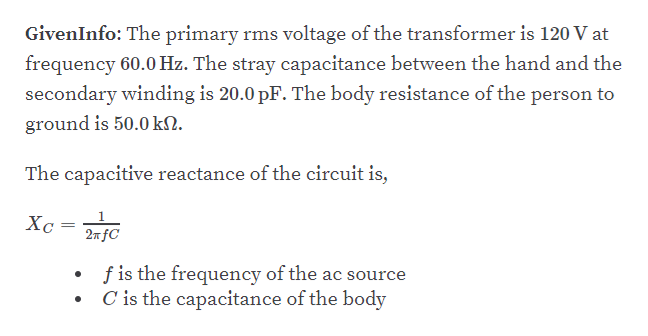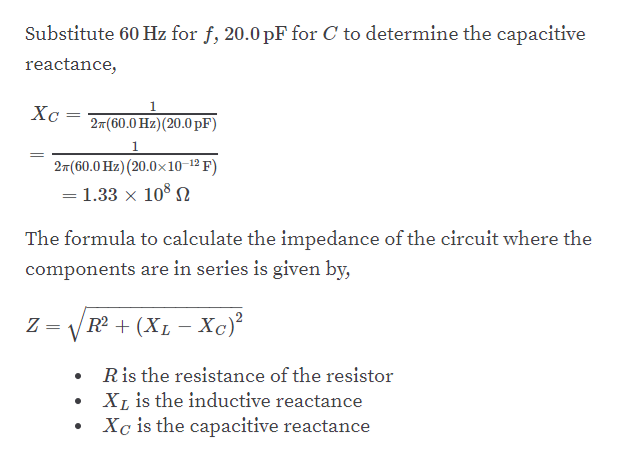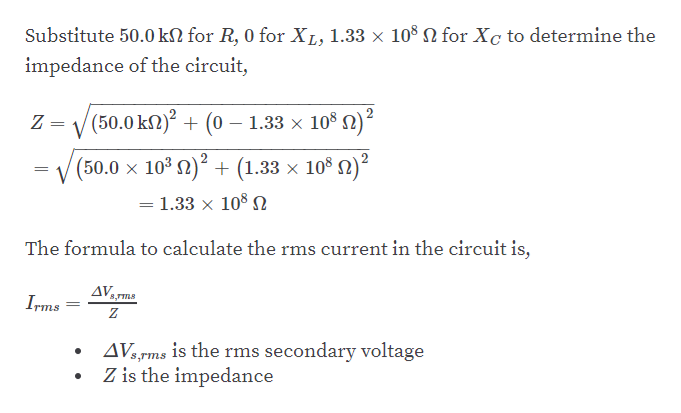Question
11 views

A person is working near the secondary of a transformer, as
shown in Figure P21.25. The primary voltage is 120. V (rms)
at 60.0 Hz. The capacitance Cs, which is the stray capacitance
between the hand and the secondary winding, is 20.0 pF.
Assuming the person has a body resistance to ground of
Rb = 50.0 kΩ, determine the rms voltage across the body.
Hint: Redraw the circuit with the secondary of the transformer
as a simple AC source.

check_circle

Step 1help_outlineImage TranscriptioncloseGivenInfo: The primary rms voltage of the transformer is 120 V at frequency 60.0 Hz. The stray capacitance between the hand and the secondary winding is 20.0 pF. The body resistance of the person to ground is 50.0 kN. The capacitive reactance of the circuit is, Xc 2n fC f is the frequency of the ac source C is the capacitance of the body fullscreen
Step 2help_outlineImage TranscriptioncloseSubstitute 60 Hz for f, 20.0 pF for C to determine the capacitive reactance, Xc 27(60.0 Hz)(20.0 pF) 1 27(60.0 Hz) (20.0x10-12 F) = 1.33 × 10° SN The formula to calculate the impedance of the circuit where the components are in series is given by, Z = V R + (XL– Xc)² Ris the resistance of the resistor XL is the inductive reactance Xc is the capacitive reactance fullscreen
Step 3help_outlineImage TranscriptioncloseSubstitute 50.0 kN for R, 0 for XL, 1.33 × 108 N for Xc to determine the impedance of the circuit, V (50.0 kN)? + (0 – 1.33 x 10° N)? = V (50.0 x 103n)² + (1.33 x 10® 2)² = 1.33 x 10° N The formula to calculate the rms current in the circuit is, AVrms 8,TTnS Irms AV;rms is the rms secondary voltage Z is the impedance fullscreen

### Want to see the full answer?

See Solution

#### Want to see this answer and more?

Solutions are written by subject experts who are available 24/7. Questions are typically answered within 1 hour.*

See Solution
*Response times may vary by subject and question.
Tagged in

### Alternating Current